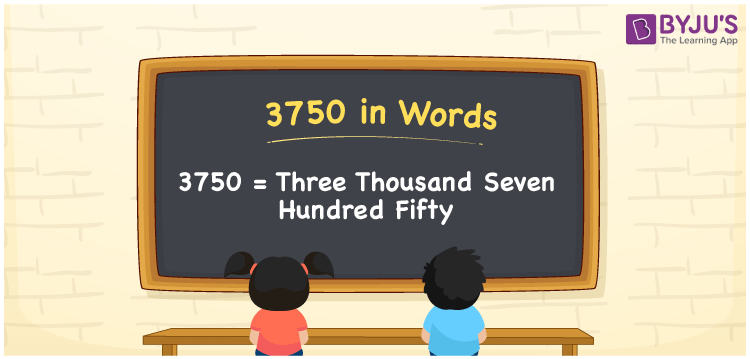# 3750 in Words

The number 3750 in words is three thousand seven hundred fifty. For converting the number 3750 into words, the place value system plays a major role. Hence, we can say that the number names must be written using a place value table. For example, if you have invested Rs. 3750 on purchasing a table, you can write “I have invested Rs. Three thousand seven hundred fifty on purchasing a table”. In this article, we will discuss the spelling of 3750 in English words and its procedure in detail.

 3750 in Words: Three Thousand Seven Hundred Fifty. Three Thousand Seven Hundred Fifty in Numerical Form: 3750.

## 3750 in English Words## How to Write 3750 in Words?

Below is the place value table for the numeral 3750:

 Thousands Hundreds Tens Ones 3 7 5 0

The expanded form of 3750 is as follows:

= 3 × Thousand + 7 × Hundred + 5 × Ten + 0 × One

= 3 × 1000 + 7 × 100 + 5 × 10

= 3000 + 700 + 50

= 3750

= three thousand seven hundred fifty

Hence, 3750 in words is three thousand seven hundred fifty.

3750 in words – Three thousand seven hundred fifty

Is 3750 an odd number? – No

Is 3750 an even number? – Yes

Is 3750 a perfect square number? – No

Is 3750 a perfect cube number? – No

Is 3750 a prime number? – No

Is 3750 a composite number? – Yes

## Frequently Asked Questions on 3750 in Words

### Write 3750 in words.

3750 in words is three thousand seven hundred fifty.

### Simplify 3000 + 750, and express in words.

Simplifying 3000 + 750, we get 3750. Hence, 3750 in words is three thousand seven hundred fifty.

### How to write three thousand seven hundred fifty in numbers?

Three thousand seven hundred fifty in numbers is 3750.